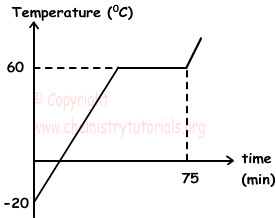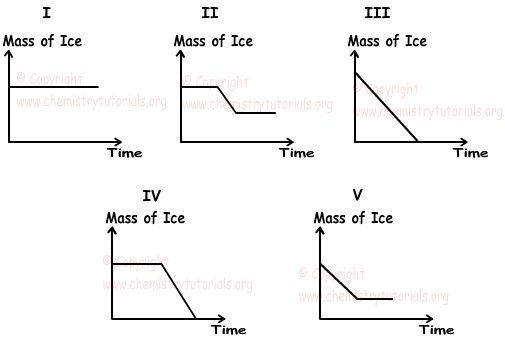EN | ES | DE | NL | RU

## Matters and Properties of Matters Exam4 and Problem Solutions

Matters and Properties of Matters Exam4 and  Problem Solutions

1. Phase diagram of X is given below. Using this diagram, find which ones of the following statements are true ?I. At 85 0C, if we decrease pressure of matter under 1 atm, it can change state liquid to gas.

II. Matter can sublimate under 0,6 atm pressure.

III. Decreasing atmosphere pressure decreases freezing point of matter.

Solution:

At 85 0C and 1 atm pressure matter exists in gas and liquid states. If pressure decreases, then matter change states into gas. I is true

0,6 atm pressure is at triple point. Under this value, if matter is heated than it can sublimate. II is true

As you can see from the diagram, solid-liquid line shows that, decreasing in pressure decreases freezing point. III is true

2. Graph given below shows heating of 10 g pure X matter at -20 0C, by heater giving heat 40 cal per minute.If specific heat capacity of  X is 0,5 cal/g 0C, find latent heat of vaporization of this matter.

Solution:

Heat gained during 75 minutes;

Q= 40 cal/minute.75minutes=3000 cal

Heat necessary for increase temperature from -20 0C to 60 0C

Q1=m.c.ΔT=(10).(0,5).(60-(-20))=400 cal

Heat necessary for vaporization is;

Q2=m.Lvap=10.Lvap

Q=Q1+Q2

3000 = 400 + Q2

Q2=2600 cal

Lvap=2600/10=260 cal/g

3. If we mix ice and water at different temperatures, which one of the following graphs does not show change in the mass of ice.Solution:

Since temperatures of water and ice are different, there is heat transfer between them. Thus, mass of ice must change. In first graph, mass of ice is constant, so it is false.

4. Pure water boils at 100 0C at 1 atm pressure. To decrease boiling point of water, which ones of following statements should be applied?

I. Dissolve salt in water

II. Decrease atmosphere pressure

III. Increase capacity of heater

Solution:

Boiling point depends on types of matter, atmospheric pressure and purity of matter. Capacity of heater does not affect boiling point. Dissolving salt in water increase boiling point of water. On the contrary, decreasing atmosphere pressure decreases boiling point of water.

5. Which ones of the following quantities change with amount of matter?

I. Density

II. Volume

III. Melting point

Solution:

Density is constant under constant temperature and pressure.

Volume increases with increasing mass and decreases with decreasing mass.

Melting point is not affected by amount of matter.

II changes with amount of matter.

The Original Author: